Latest Banking jobs   »

# Reasoning Ability Quiz For IBPS SO Prelims 2022- 13th December

Direction (1-5): Study the following number series carefully and answer the questions accordingly.

423 587 267 386 845 665

Q1. If the last digit of each number is multiplied by 3 then what will be the sum of all the third digits within the numbers after multiplication?
(a) 94
(b) 104
(c) 114
(d) 99
(e) None of these

Q2. If we subtract 2 from the second digit of each number and add 1 to the first digit of each number, then which of the following will be the third highest number?
(a) 423
(b) 665
(c) 587
(d) 386
(e) None

Q3. If the first two digits of each number are interchanged then what will be the resultant of the sum of the lowest and the second highest number?
(a) 1132
(b) 1079
(c) 1010
(d) 1099
(e) None of these

Q4. If all the digits in each number are written in ascending order within the number, then which of the given number will become the second highest?
(a) 587
(b) 386
(c) 665
(d) 845
(e) None of these

Q5. If the first two digits of each number are interchanged then which of the following number will be second highest?
(a) 267
(b) 386
(c) 423
(d) 587
(e) None of these

Q6. If in the word ‘PRODUCE’, all the vowels are changed to the immediate next letter and all the consonants are changed to the immediate previous letter. Which of the following letters is fourth from the right end?
(a) P
(b) O
(c) V
(d) C
(e) None of these

Q7. How many such pairs of letters are there in the word ‘SEGMENT’ each of which has as many letters between them in the word as in the English alphabet (Both forward and backward)?
(a) One
(b) Two
(c) Three
(d) More than three
(e) None

Q8. How many letters appear twice by changing the consonants and the vowels in the word ‘PARTICLE’ when each of the consonants is replaced by the immediate previous letter in the English alphabet and the vowels are replaced by the immediate next letter in the English alphabet?
(a) One
(b) Two
(c) Three
(d) More than three
(e) None

Q9. How many such pairs of letters are there in the word ‘DISPENSE’ each of which has as many letters between them in the word as in the English alphabet (both forward and backward)?
(a) One
(b) Two
(c) Three
(d) More than three
(e) None

Q10. If it is possible to make a meaningful word from 3rd, 4th, 5th and 9th letters of the word TURPITUDE, then what is the third letter from the left of the word formed? Mark M if no such word can be formed and mark N if more than one such word can be formed.
(a) R
(b) E
(c) P
(d) M
(e) N

Direction (11-13): Study the following information and answer the given questions.
Siara starts from point A and moves towards the north and covers a distance of 50m to reach point B then turns east and covers a distance of 80m to reach point C then turns south and covers a distance of 50m to reach point D and turns west and covers a distance of 30m to reach finally at point E.

Q11. What is the shortest distance between initial and final position of Siara?
(a) 30m
(b) 40m
(c) 50m
(d) 60m
(e) None of these

Q12. Point C is in which direction with respect to point A?
(a) North-west
(b) North-east
(c) West
(d) South
(e) North

Q13. What is the shortest distance between point A and point D?
(a) 50m
(b) 30m
(c) 40m
(d) 80m
(e) None of these

Direction (14-15): Study the following information carefully and answer the questions accordingly.
Mahesh starts walking from point C to reach point B which is 30m west of point C. Then he takes a left turn and walks 40m to reach point E. From E, he takes a right turn and walks 30m to reach point F. Then, again he took a right turn and walks 20m to reach point G. From point G, he takes a right turn and walks 40m to reach point H.

Q14. Four among the following five are same in a certain way and related to a group. Which among the following does not belong to the group?
(a) B, G
(b) G, C
(c) C, H
(d) B, F
(e) H, E

Q15. If point J is 20m south of point H then what is the shortest distance between F and J?
(a) 32m
(b) 46m
(c) 35m
(d) 40m
(e) None of these

Solutions

S1. Ans. (d)
Sol. The required sum = (3 x 3) + (7 x 3) + (7 x 3) + (6 x 3) + (5 x 3) + (5 x 3) = 99
Hence, the required answer is ‘99’.

S2. Ans. (c)
Sol. After addition and subtraction – 503 667 347 466 925 745
Hence, the required answer is ‘587’.

S3. Ans. (b)
Sol. After interchange – 243 857 627 836 485 665
The required sum – 836 + 243 = 1079

S4. Ans. (c)
Sol. After writing in ascending order – 234 578 267 368 458 566
Hence, the required answer is ‘665’.

S5 Ans. (b)
Sol. After interchange – 243 857 627 836 485 665
Hence, the required answer is ‘386’.

S6. Ans. (d)
Sol.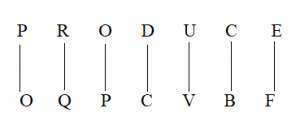S7. Ans. (b)
Sol.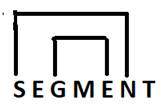S8. Ans. (a)
Sol. Given Word – PARTICLE
After the rearrangement PARTICLE – OBQSJBKF

S9. Ans. (b)
Sol.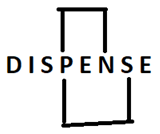S10. Ans. (e)
Sol. The meaningful word from 3rd, 4th, 5th and 9th letters of the word TURPITUDE is “RIPE” & “PIER”.

Solution (11-13):
Sol.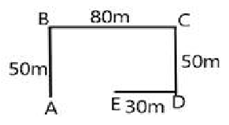S11. Ans. (c)
S12. Ans. (b)
S13. Ans. (d)

Solution (14-15):
Sol.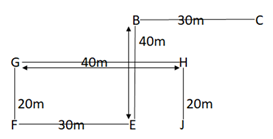S14. Ans. (b)
S15. Ans. (d)#### Congratulations!Union Budget 2023-24: Free PDF